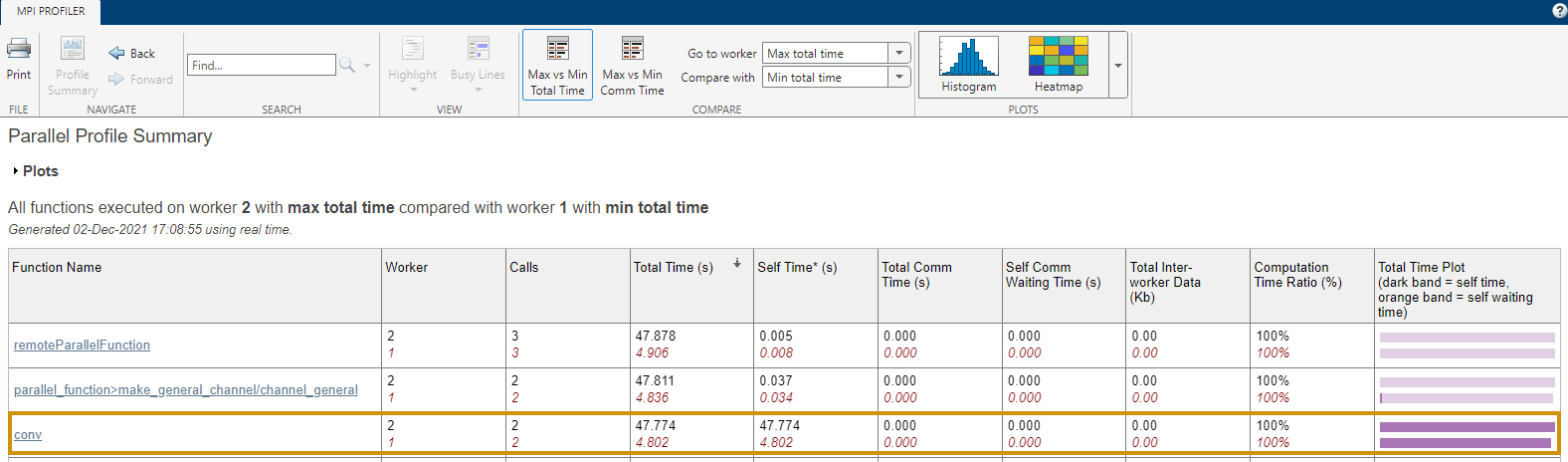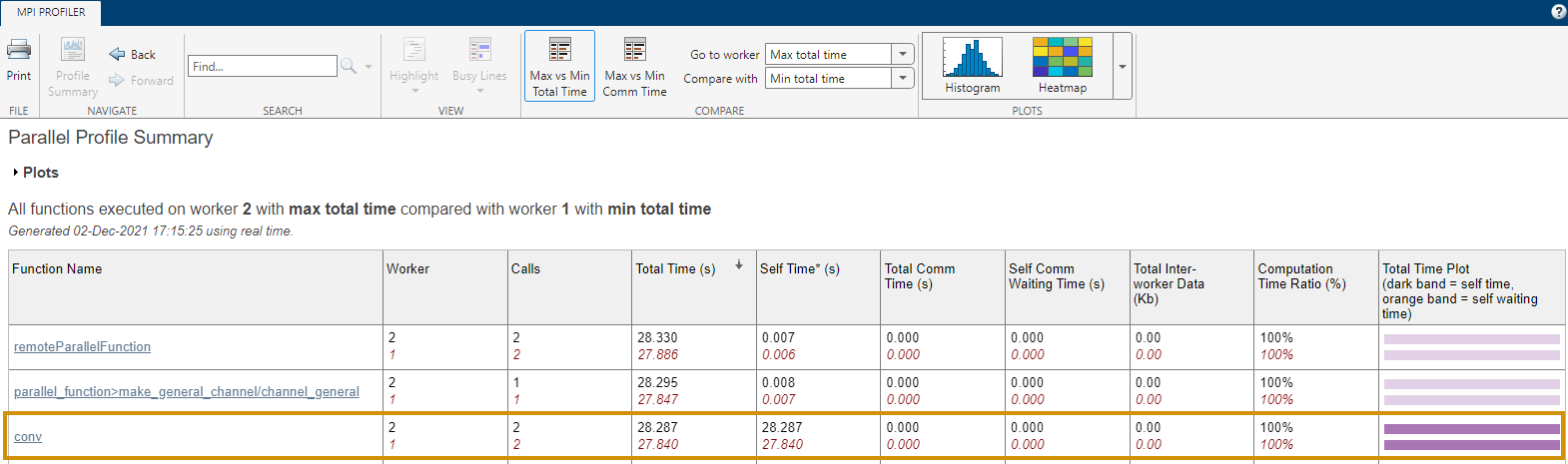# Profile Parallel Code

This example shows how to profile parallel code using the parallel profiler on workers in a parallel pool.

Create a parallel pool.

```numberOfWorkers = 3; pool = parpool(numberOfWorkers);```
```Starting parallel pool (parpool) using the 'local' profile ... Connected to the parallel pool (number of workers: 3). ```

Collect parallel profile data by enabling `mpiprofile`.

`mpiprofile on`

Run your parallel code. For the purposes of this example, use a simple `parfor` loop that iterates over a series of values.

```values = [5 12 13 1 12 5]; tic; parfor idx = 1:numel(values) u = rand(values(idx)*3e4,1); out(idx) = max(conv(u,u)); end toc```
```Elapsed time is 51.886814 seconds. ```

After the code completes, view the results from the parallel profiler by calling `mpiprofile viewer`. This action also stops profile data collection.

`mpiprofile viewer`

The report shows execution time information for each function that runs on the workers. You can explore which functions take the most time in each worker.

Generally, comparing the workers with the minimum and maximum total execution times is useful. To do so, click Max vs Min Total Time in the report. In this example, observe that `conv` executes multiple times and takes significantly longer in one worker than in the other. This observation suggests that the load might not be distributed evenly across the workers.• If you do not know the workload of each iteration, then a good practice is to randomize the iterations, such as in the following sample code.

```values = values(randperm(numel(values))); ```
• If you do know the workload of each iteration in your `parfor` loop, then you can use `parforOptions` to control the partitioning of iterations into subranges for the workers. For more information, see `parforOptions`.

In this example, the greater `values(idx)` is, the more computationally intensive the iteration is. Each consecutive pair of values in `values` balances low and high computational intensity. To distribute the workload better, create a set of `parfor` options to divide the `parfor` iterations into subranges of size `2`.

`opts = parforOptions(pool,"RangePartitionMethod","fixed","SubrangeSize",2);`

Enable the parallel profiler.

`mpiprofile on`

Run the same code as before. To use the `parfor` options, pass them to the second input argument of `parfor`.

```values = [5 12 13 1 12 5]; tic; parfor (idx = 1:numel(values),opts) u = rand(values(idx)*3e4,1); out(idx) = max(conv(u,u)); end toc```
```Elapsed time is 33.813523 seconds. ```

Visualize the parallel profiler results.

`mpiprofile viewer`

In the report, select Max vs Min Total Time to compare the workers with the minimum and maximum total execution times. Observe that this time, the multiple executions of `conv` take a similar amount of time in all workers. The workload is now better distributed.